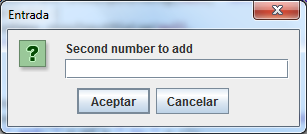# User interface input data validation.

In this tutorial we will develop the validation for the data inserted by the user. In the example below we add the two numbers given by the user. To do so, we first have to verify that the user has inserted two numbers and not any other character, which can´t be add up.

In the first example "Adding1", we ask the user to introduce two numbers in our InputDialog boxes:```package com.edu4java.swing.tutrial2;

import javax.swing.JOptionPane;

public static void main(String[] args) {
String n1 = JOptionPane.showInputDialog(null, "First Number");
String n2 = JOptionPane.showInputDialog(null, "Second Number");

int r = new Integer(n1) + new Integer(n2);

JOptionPane.showMessageDialog(null, "the result is " + r);
}
}

```

If the user inserts both numbers correctly there will be no problem. But if the user inserts any other character, like a "h" for example, there will be an error. To avoid this, we develop an input data validation as we can see in the class "Adding2":

```package com.edu4java.swing.tutrial2;

import javax.swing.JOptionPane;

public static void main(String[] args) {
String n1 = JOptionPane.showInputDialog(null, "First number to add");
while (!isNumber(n1)) {
n1 = JOptionPane.showInputDialog(null,
"Invalid first number. Please insert another number");
}
String n2 = JOptionPane.showInputDialog(null, "Second number to add");
while (!isNumber(n2)) {
n2 = JOptionPane.showInputDialog(null,
"Invalid second number. Please insert another number");

}

int r = new Integer(n1) + new Integer(n2);
JOptionPane.showMessageDialog(null, "The result of the addition of: "
+ n1 + " and: " + n2 + " is " + r);

}

private static boolean isNumber(String n) {
try {
Integer.parseInt(n);
return true;
} catch (NumberFormatException nfe) {
return false;
}
}
}

```

If the user inserts a character which is not a number, we inform him that he is not inserting a valid data and that he has to insert a new number. This will be repeated until the user inserts a number. For this we will use the "while" sentence. Firstly, with the first number and then with the second number. Once we have the both numbers validated, we add them up.

 << Previous Next >>